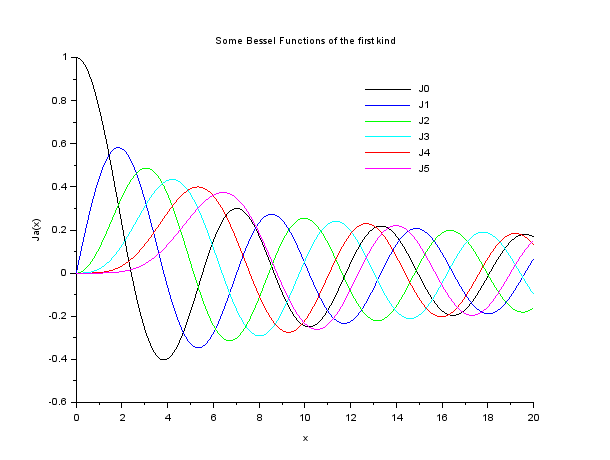# Plotting the First 6 Bessel Functions(Jn(x)) of First Kind in SCILAB

Scilab comes pre-loaded with a variety of functions to handle the Bessel and Modified Bessel Functions of First and Second Kind.

These are:

#### besseli

Modified Bessel functions of the first kind (I sub alpha).

#### besselj

Bessel functions of the first kind (J sub alpha).

#### besselk

Modified Bessel functions of the second kind (K sub alpha).

#### bessely

Bessel functions of the second kind (Y sub alpha).

#### besselh

Bessel functions of the third kind (aka Hankel functions)

### Calling Sequence

y = besseli(alpha,x [,ice])
y = besselj(alpha,x [,ice])
y = besselk(alpha,x [,ice])
y = bessely(alpha,x [,ice])
y = besselh(alpha,x)
y = besselh(alpha,K,x [,ice])

### Arguments

x

real or complex vector.

alpha

real vector

ice

integer flag, with default value 0

K

integer, with possible values 1 or 2, the Hankel function type.

Now our objective is to plot the First 6 Bessel Functions of the First Kind.
So I am going to use besselj(alpha,x) .

The following code which is pretty much self-explanatory calculates and plots the First six Bessel Functions, that is J0(x), J1(x), J2(x),…,J5(x).

```//Bessel Functions of the First Kind
//Plotting some Bessel Functions
clf;
x=[0:0.01:20]';
alpha=0:5;
y=besselj(alpha,x);
plot2d(x,y,leg='[email protected]@[email protected]@[email protected]');
xlabel('x');
ylabel('Ja(x)');
xtitle('Some Bessel Functions of the first kind');```Video Tutorials: